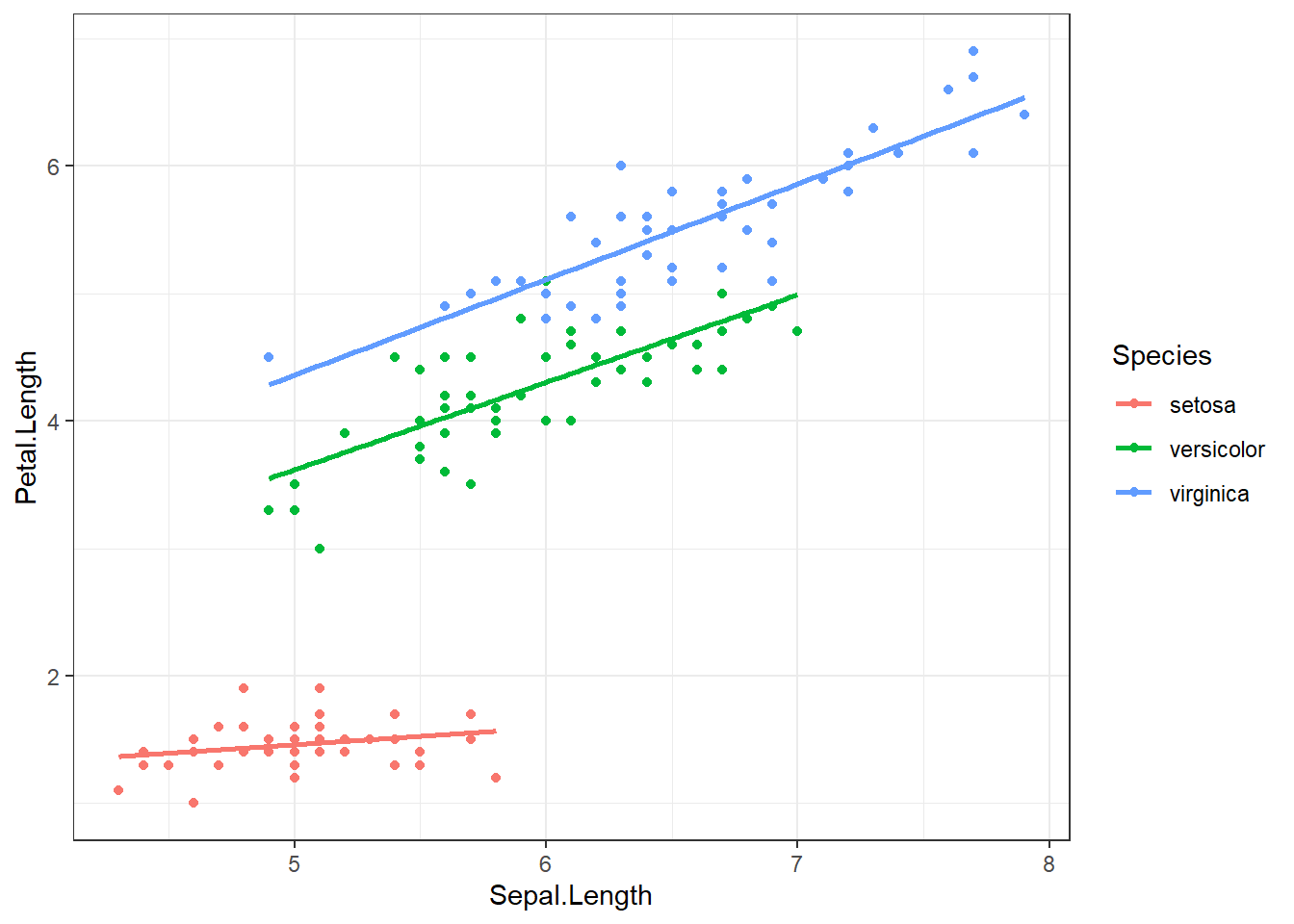## 9.1 Stratification

Stratified models examine the regression equations for each subgroup of the population and seeing if the relationship between the response and explanatory variables changed for at least one subgroup.

Consider the relationship between the length of an iris petal, and the length of it’s sepal. Earlier we found that the iris species modified this relationship. Lets consider a binary indicator variable for species that groups veriscolor and virginica together.

iris$setosa <- ifelse(iris$Species=="setosa", 1, 0)
table(iris$setosa, iris$Species)
##
##     setosa versicolor virginica
##   0      0         50        50
##   1     50          0         0

Within the setosa species, there is little to no relationship between sepal and petal length. For the other two species, the relationship looks still significantly positive, but in the combined sample there appears to be a strong positive relationship (blue).

ggplot(iris, aes(x=Sepal.Length, y=Petal.Length, col=as.factor(setosa))) +
geom_point() + theme_bw() + theme(legend.position="top") +
scale_color_manual(name="Species setosa", values=c("red", "darkgreen")) +
geom_smooth(se=FALSE, method="lm") +
geom_smooth(aes(x=Sepal.Length, y=Petal.Length), col="blue", se=FALSE, method='lm')The mathematical model describing the relationship between Petal length ($$Y$$), and Sepal length ($$X$$), for species setosa ($$s$$) versus not-setosa ($$n$$), is written as follows:

$Y_{is} \sim \beta_{0s} + \beta_{1s}*x_{i} + \epsilon_{is} \qquad \epsilon_{is} \sim \mathcal{N}(0,\sigma^{2}_{s})$ $Y_{in} \sim \beta_{0n} + \beta_{1n}*x_{i} + \epsilon_{in} \qquad \epsilon_{in} \sim \mathcal{N}(0,\sigma^{2}_{n})$

In each model, the intercept, slope, and variance of the residuals can all be different. This is the unique and powerful feature of stratified models. The downside is that each model is only fit on the amount of data in that particular subset. Furthermore, each model has 3 parameters that need to be estimated: $$\beta_{0}, \beta_{1}$$, and $$\sigma^{2}$$, for a total of 6 for the two models. The more parameters that need to be estimated, the more data we need.